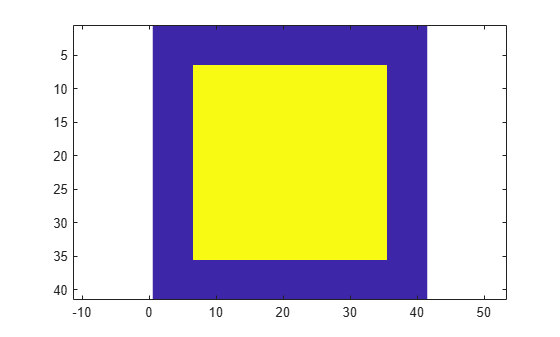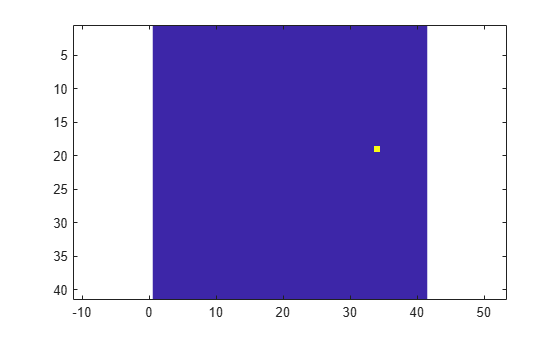# step

System object: phased.CFARDetector2D
Package: phased

Two-dimensional CFAR detection

## Syntax

``Y = step(detector,X,cutidx)``
``Y = step(detector,X,cutidx,K)``
``[Y,th] = step(___)``
``[Y,noise] = step(___)``

## Description

### Note

Alternatively, instead of using the `step` method to perform the operation defined by the System object™, you can call the object with arguments, as if it were a function. For example, ```y = step(obj,x)``` and `y = obj(x)` perform equivalent operations.

example

````Y = step(detector,X,cutidx)` performs 2-D CFAR detection on input image data, `X`, for the image cells under test (CUT) specified by `cutidx`. `Y` contains the detection results for the CUT cells.```
````Y = step(detector,X,cutidx,K)` also specifies a threshold factor, `K`, for setting the detection threshold. This syntax applies when the `ThresholdFactor` property of the detector is set to `'Input port'`.```

example

````[Y,th] = step(___)` also returns the detection threshold, `th`, applied to detected cells under test. To enable this syntax, set the `ThresholdOutputPort` property to `true`.```

example

````[Y,noise] = step(___)` also returns the estimated noise power, `noise`, applied to detected cells under test. To enable this syntax, set the `NoisePowerOutputPort` property to `true`.```

### Note

The object performs an initialization the first time the object is executed. This initialization locks nontunable properties (MATLAB) and input specifications, such as dimensions, complexity, and data type of the input data. If you change a nontunable property or an input specification, the System object issues an error. To change nontunable properties or inputs, you must first call the `release` method to unlock the object.

## Input Arguments

expand all

Two-dimensional CFAR detector, specified as a `phased.CFARDetector2D` System object.

Input image, specified as a real M-by-N matrix or a real M-by-N-by-P array. M and N represent the rows and columns of a matrix. Each page is an independent 2-D signal.

The size of the first dimension of the input matrix can vary to simulate a changing signal length. A size change can occur, for example, in the case of a pulse waveform with variable pulse repetition frequency.

Example: `[1,1;2.5,1;0.5,0.1]`

Data Types: `single` | `double`

Test cells, specified as a 2-by-D matrix of positive integers, where D is the number of test cells. Each column of `cutidx` specifies the row and column indices of a CUT cell. The same indices apply to all pages in the input array. You must restrict the locations of CUT cells so that their training regions lie completely within the input images.

Example: `[10,15;11,15;12,15]`

Data Types: `single` | `double`

Threshold factor used to calculate the detection threshold, specified as a positive scalar.

#### Dependencies

To enable this input argument, set the `ThresholdFactor` property of the detector object to `'Input port'`

Data Types: `single` | `double`

## Output Arguments

expand all

Detection results, whose format depends on the `OutputFormat` property

• When `OutputFormat` is ```'Cut result'```, `Y` is a D-by-P matrix containing logical detection results for cells under test. D is the length of `cutidx` and P is the number of pages of `X`. The rows of `Y` correspond to the rows of `cutidx`. For each row, `Y` contains `1` in a column if there is a detection in the corresponding cell in `X`. Otherwise, `Y` contains a `0`.

• When `OutputFormat` is ```'Detection report'```, `Y` is a K-by-L matrix containing detections indices. K is the number of dimensions of `X`. L is the number of detections found in the input data. When `X` is a matrix, `Y` contains the row and column indices of each detection in `X` in the form `[detrow;detcol]`. When `X` is an array, `Y` contains the row, column, and page indices of each detection in `X` in the form `[detrow;detcol;detpage]`. When the `NumDetectionsSource` property is set to `'Property'`, L equals the value of the `NumDetections` property. If the number of actual detections is less than this value, columns without detections are set to `NaN`.

Data Types: `single` | `double`

Computed detection threshold for each detected cell, returned as a real-valued matrix. `Th` has the same dimensions as `Y`.

• When `OutputFormat` is ```'CUT result'```, `Th` returns the detection threshold whenever an element of `Y` is `1` and `NaN` whenever an element of `Y` is `0`.

• When `OutputFormat` is ```'Detection index'```, `th` returns a detection threshold for each corresponding detection in `Y`. When the `NumDetectionsSource` property is set to `'Property'`, L equals the value of the `NumDetections` property. If the number of actual detections is less than this value, columns without detections are set to `NaN`.

#### Dependencies

To enable this output argument, set the `ThresholdOutputPort` to `true`.

Data Types: `single` | `double`

Estimated noise power for each detected cell, returned as a real-valued matrix. `noise` has the same dimensions as `Y`.

• When `OutputFormat` is ```'CUT result'```, `noise` returns the noise power whenever an element of `Y` is `1` and `NaN` whenever an element of `Y` is `0`.

• When `OutputFormat` is ```'Detection index'```, `noise` returns a noise power for each corresponding detection in `Y`. When the `NumDetectionsSource` property is set to `'Property'`, L equals the value of the `NumDetections` property. If the number of actual detections is less than this value, columns without detections are set to `NaN`.

#### Dependencies

To enable this output argument, set the `NoisePowerOutputPort` to `true`.

Data Types: `single` | `double`

## Examples

expand all

This example shows how to set a 2-D CFAR threshold based upon a required probability of false alarm (pfa).

Note: You can replace each call to the function with the equivalent `step` syntax. For example, replace `myObject(x)` with `step(myObject,x)`.

Perform cell-averaging CFAR detection on a 41-by-41 matrix of cells containing Gaussian noise. Estimate the empirical pfa and compare it to the required pfa. To get a good estimate, perform this simulation on 1000 similar matrices. First, set a threshold using the required pfa. In this case, there are no targets and the pfa can be estimated from the number of cells that exceed the threshold. Assume that the data is processed through a square-law detector and that no pulse integration is performed. Use a training-cell band of 3 cells in width and 4 cells in height. Use a guard band of 3 cells in width and 2 cells in height to separate the cells under test (CUT) from the training cells. Specify a required pfa of 5.0e-4.

```p = 5e-4; rs = RandStream.create('mt19937ar','Seed',5); N = 41; ntrials = 1000; detector = phased.CFARDetector2D('TrainingBandSize',[4,3], ... 'ThresholdFactor','Auto','GuardBandSize',[2,3], ... 'ProbabilityFalseAlarm',p,'Method','SOCA','ThresholdOutputPort',true);```

Create a 41-by-41 image containing random complex data. Then, square the data to simulate a square-law detector.

```x = 2/sqrt(2)*(randn(rs,N,N,ntrials) + 1i*randn(rs,N,N,ntrials)); x2 = abs(x).^2;```

Process all the cells in each image. To do this, find the row and column of each CUT cell whose training region falls entirely within each image.

```Ngc = detector.GuardBandSize(2); Ngr = detector.GuardBandSize(1); Ntc = detector.TrainingBandSize(2); Ntr = detector.TrainingBandSize(1); cutidx = []; colstart = Ntc + Ngc + 1; colend = N - ( Ntc + Ngc); rowstart = Ntr + Ngr + 1; rowend = N - ( Ntr + Ngr); for m = colstart:colend for n = rowstart:rowend cutidx = [cutidx,[n;m]]; end end ncutcells = size(cutidx,2);```

Display the CUT cells.

```cutimage = zeros(N,N); for k = 1:ncutcells cutimage(cutidx(1,k),cutidx(2,k)) = 1; end imagesc(cutimage) axis equal```Perform the detection on all CUT cells. Return the detection classification and the threshold used to classify the cell.

`[dets,th] = detector(x2,cutidx);`

Find and display an image with a false alarm for illustration.

```di = []; for k = 1:ntrials d = dets(:,k); if (any(d) > 0) di = [di,k]; end end idx = di(1); detimg = zeros(N,N); for k = 1:ncutcells detimg(cutidx(1,k),cutidx(2,k)) = dets(k,idx); end imagesc(detimg) axis equal```Compute the empirical pfa.

`pfa = sum(dets(:))/ntrials/ncutcells`
```pfa = 4.5898e-04 ```

The empirical and specified pfa agree.

Display the average empirical threshold value over all images.

`mean(th(:))`
```ans = 31.7139 ```

Compute the theoretical threshold factor for the required pfa.

```threshfactor = npwgnthresh(p,1,'noncoherent'); threshfactor = 10^(threshfactor/10); disp(threshfactor)```
``` 7.6009 ```

The theoretical threshold factor multiplied by the noise variance should agree with the measured threshold.

```noisevar = mean(x2(:)); disp(threshfactor*noisevar);```
``` 30.4118 ```

The theoretical threshold and empirical threshold agree to within an acceptable difference.

Perform cell-averaging CFAR detection on a 41-by-41 matrix of cells containing five closely-spaced targets in Gaussian noise. Perform this detection on a simulation of 1000 images. Use two detectors with different guard band regions. Set the thresholds manually using the `Custom` threshold factor. Assume that the data is processed through a square law-detector and that no pulse integration is performed. Use a training cell band of 2 cells in width and 2 cells in height. For the first detector, use a guard band of 1 cell all around to separate the CUT cells from the training cells. For the second detector, use a guard band of 8 cells all around.

Note: This example runs only in R2016b or later. If you are using an earlier release, replace each call to the function with the equivalent `step` syntax. For example, replace `myObject(x)` with `step(myObject,x)`.

```p = 5e-4; rs = RandStream.create('mt19937ar','Seed',5); N = 41; ntrials = 1000;```

Create 1000 41-by-41 images of complex random noise with standard deviation of 1.

```s = 1; x = s/sqrt(2)*(randn(rs,N,N,ntrials) + 1i*randn(rs,N,N,ntrials));```

Set the target cells values to 1.5. Then, square the cell values.

```A = 1.5; x(23,20,:) = A; x(23,18,:) = A; x(23,23,:) = A; x(20,22,:) = A; x(21,18,:) = A; x2 = abs(x).^2;```

Display the target cells.

```xtgt = zeros(N,N); xtgt(23,20,:) = A; xtgt(23,18,:) = A; xtgt(23,23,:) = A; xtgt(20,22,:) = A; xtgt(21,18,:) = A; imagesc(xtgt) axis equal axis tight```Set the CUT cells to be the target cells.

```cutidx(1,1) = 23; cutidx(2,1) = 20; cutidx(1,2) = 23; cutidx(2,2) = 18; cutidx(1,3) = 23; cutidx(2,3) = 23; cutidx(1,4) = 20; cutidx(2,4) = 22; cutidx(1,5) = 21; cutidx(2,5) = 18;```

Perform the detection on all CUT cells using two CFAR 2-D detectors. The first detector has a small guard band region. The training region can include neighboring targets which can affect the computation of the noise power. The second detector has a larger guard band region, which precludes target cells from being used in the noise computation.

Create the two CFAR detectors.

```detector1 = phased.CFARDetector2D('TrainingBandSize',[2,2], ... 'GuardBandSize',[1,1],'ThresholdFactor','Custom','Method','CA', ... 'CustomThresholdFactor',2,'ThresholdOutputPort',true); detector2 = phased.CFARDetector2D('TrainingBandSize',[2,2], ... 'GuardBandSize',[8,8],'ThresholdFactor','Custom','Method','CA', ... 'CustomThresholdFactor',2,'ThresholdOutputPort',true);```

Return the detection classifications and the thresholds used to classify the cells. Then, compute the probabilities of detection.

```[dets1,th1] = detector1(x2,cutidx); ndets = numel(dets1(:)); pd1 = sum(dets1(:))/ndets```
```pd1 = 0.6416 ```
```[dets2,th2] = detector2(x2,cutidx); pd2 = sum(dets2(:))/ndets```
```pd2 = 0.9396 ```

The detector with the larger guard-band region has a higher pfa because the noise is more accurately estimated.